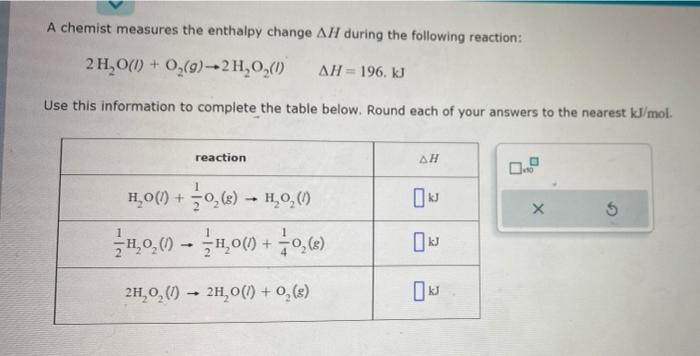Home / Expert Answers / Chemistry / a-chemist-measures-the-enthalpy-change-delta-h-during-the-following-reaction-2-mathrm-h-pa236

# (Solved): A chemist measures the enthalpy change $$\Delta H$$ during the following reaction: $2 \mathrm{H ...A chemist measures the enthalpy change $$\Delta H$$ during the following reaction: \[ 2 \mathrm{H}_{2} \mathrm{O}(I)+\mathrm{O}_{2}(g) \rightarrow 2 \mathrm{H}_{2} \mathrm{O}_{2}(\text { I } \quad \quad \Delta H=196 . \mathrm{kJ}$ Use this information to complete the table below. Round each of your answers to the nearest $$\mathrm{kJ} / \mathrm{mol}$$.

We have an Answer from Expert